{{announcement.body}}
{{announcement.title}}

# How to Perform Arithmetic Operations on Numeric Variables When Load Testing

DZone 's Guide to

# How to Perform Arithmetic Operations on Numeric Variables When Load Testing

### Testers often need to conduct arithmetic operations on variables in load or functional testing. See how to accomplish this with JMeter.

· Performance Zone ·
Free Resource

Comment (0)

Save
{{ articles.views | formatCount}} Views

When we are performing load or functional tests, we often need to conduct arithmetic operations on our variables. Imagine for example that we are testing an API that returns three parameters with a numeric value. One of the parameters is the sum of the other two. To verify that the API performs the addition operation correctly, we need to perform the addition operation ourselves and compare it to the value returned by the API.

To perform arithmetic operations on variables of a numeric data type (these are int, long, float, double, short, byte), we use the arithmetic characters shown in the table below.

In arithmetic operations with an assignment, a joint operation is performed: first adding/subtracting/etc., and then assigning the result to the variable. These constructions are quite common when using the logic control operators "while" and "for".

Let's look at an example.

The expression a + = 4, is equivalent to the following expression a = a + 4. If in the code we replace a + = 4 by a = a + 4, then we get the identical result. The table below shows the result of the + = operator at each step, if we were using a "for" loop.

In order to implement the execution of arithmetic operations in Apache JMeter™, it is necessary to do the following.

Test Plan -> Add -> Threads (Users) -> Thread Group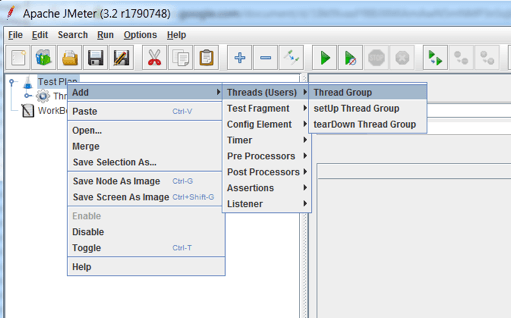Thread Group -> Add -> Sampler -> JSR223 Sampler3. Choose the language: Java (BeanShell 2.0b5/BeanShell Engine 1.0)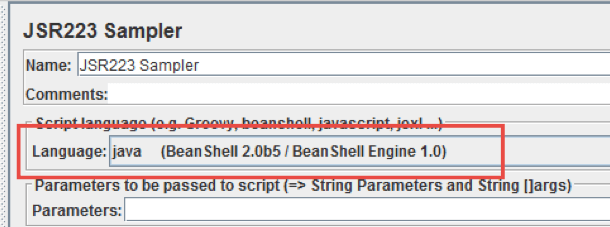4. Open the JMeter console. The JMeter console will be used to show the results of performing arithmetic operations.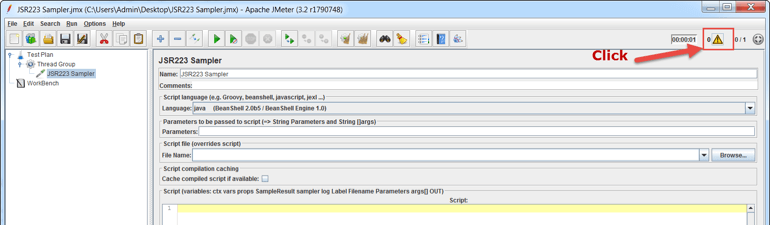5. In the JSR223 Sampler, add your code. To start out, use the following code example.

### Example Code

log.info - This is a method that outputs information to the JMeter console. In the code example above, the console displays the value of the variable . When writing large tests, it's valuable to output error messages to the console, to see if an error occurs at some step. This significantly reduces the time to find out why the error occurred.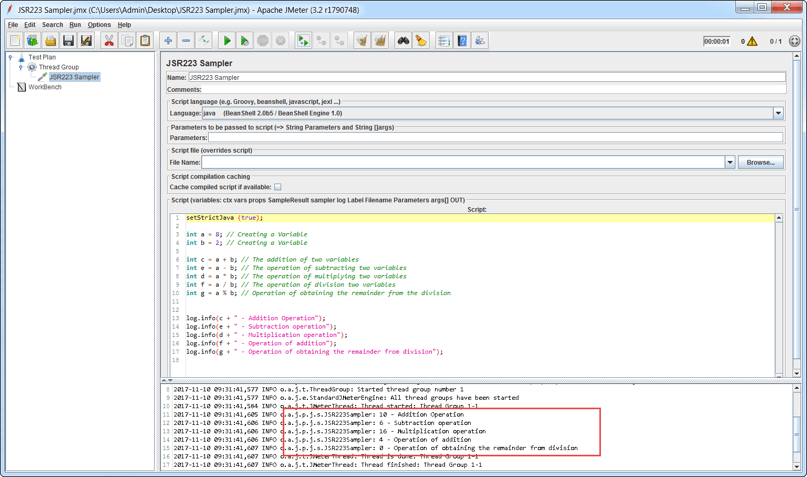In JMeter, dividing a number by 0 causes an error, as you can see in the screenshot below:

Example code: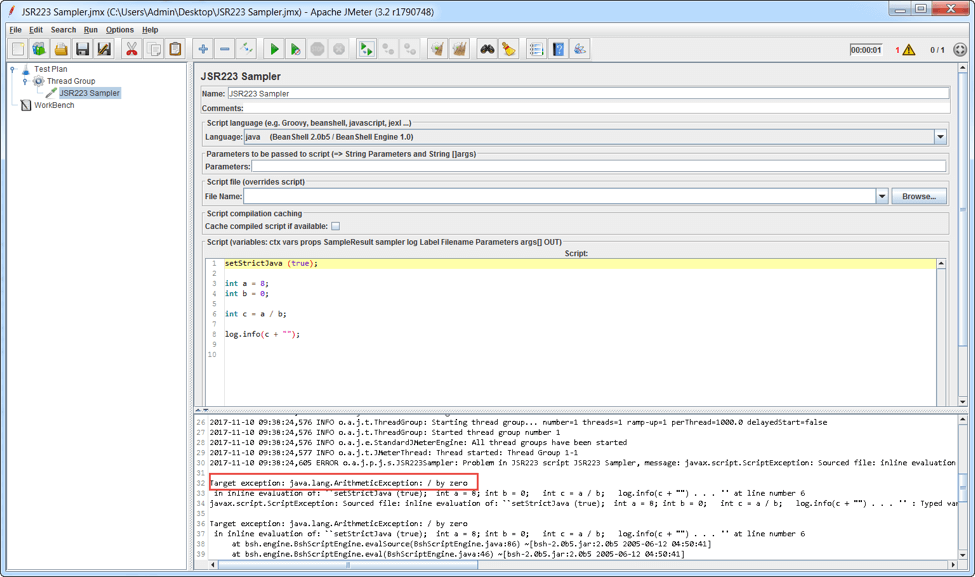Now let's look at a more advanced example.

When writing autotests, arithmetic operations with assignments are used quite often. These operations reduce the amount of written code, and also calculate in Java faster.

Example code: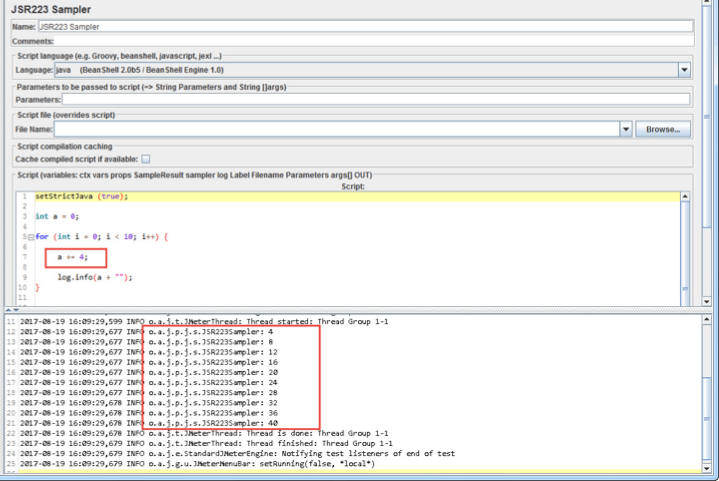The sample code demonstrates the operation of addition with assignment simultaneously. The increment operation is used. Let's consider this code in more detail.

• int a = 0 - Creating a Variable;
• for (int i = 0; i < 10; i++) - a cycle with a condition that repeats the assignment 10 times, adding 1 to i each time.
• int i = 0 - This is the initial value for calculating the number of steps in the cycle
• i < 10 - This condition, upon which the cycle will stop
• i++ - An incrementing operation that increments the value of the variable by 1.
• Log.info - the method of outputting data to the JMeter console

Topics:
apache jmeter ,api testing ,functional tests ,jmeter ,load testing ,performance ,performance testing ,variables

Comment (0)

Save
{{ articles.views | formatCount}} Views

Published at DZone with permission of Sergey Horban , DZone MVB. See the original article here.

Opinions expressed by DZone contributors are their own.